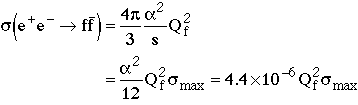(click on the above title for a GIF version of the notes)

The universe never fails, but our theories do!

The cross section for any distinguishable scattering process have a cross section less than

smax = 4p/pcm2
= 16
p/s = (19.6 mb GeV2)/s  (in high energy limit)

Examples:

(1) e+e- annihilation into point fermion-antifermion pairNo problem for muons (Qm=1) or quarks (Qu= Qc= Qt=2/3, Qd= Qs= Qb=1/3), but what if a point fermion existed with charge Qf=475?

Answer: Perturbation theory would break down, and the QED cross section would be reduced below the unitarity bound.

(2) billiard balls (or neutron-proton scattering at high energies) have a essentially constant total cross section

sbilliards = 4pR2

This violates unitarity if pcm>2/R!

Answer:: In billiard ball scattering, the number of orbital angular momentum states is proportional to s, so the constant cross section is maintained because the number of scattering states increases at a rate which cancels out the reduction in the maximum cross section per scattering state.

(3) neutrino-proton interactions

s(nmp) = 0.4x10-38cm2/GeV2 = smax s2/(150 GeV)4

This cross section formula cannot be true for c.o.m. energies above 150 GeV!

A Yukawa force mediated by a massive particle

V(x) = (-g2/x) exp(-xMB)

gives a cross section

s = 4pg4s/MB4

in the low energy limit. In the high energy limit (corresponding to x very small) this potential is just a colomb-like potential and the cross section turns over and falls as 1/s just like the e+e- annihilation cross-section. The boson must have a mass less than 150 GeV (Prediction!), so that the turn-over occurs before the unitarity violation would occur.

(4) WW scattering violates unitarity at about 1.5 TeV

Theoretical solution: Higgs or supersymmetry or W substructure or …

Experimental solution: Build the biggest accelerator you can convince your government(s) to afford (SSC - nope, LHC yes), and see what happens.